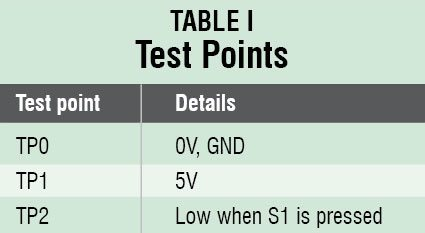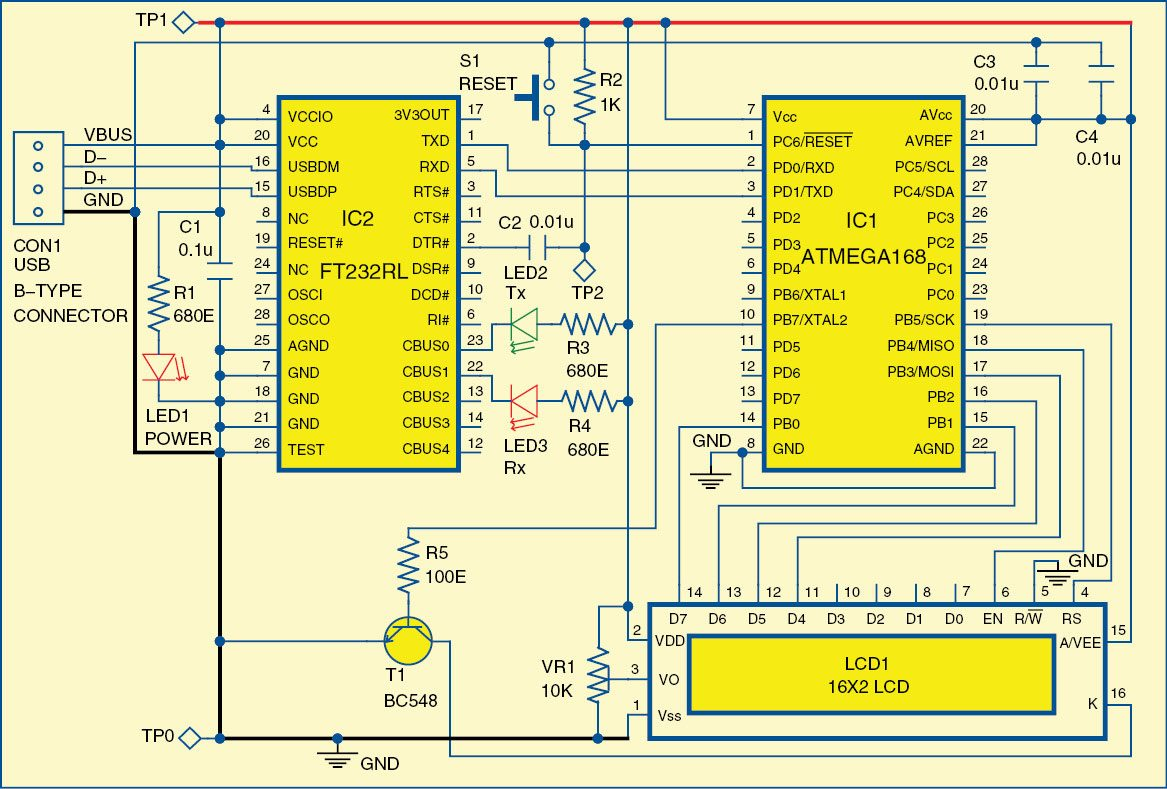Tuesday, August 16, 2022

# Calculator Using Postfix Notation

By T. Anand. He is a co-founder of Knewron Technologies, has over 12 years of direct experience with small-, medium- and large-scale projects in manufacturing, service sectors, electronics and electrical systems, IT, etc. A chartered engineer by profession, he also has broad experience in R&D of IT, white goods, electronic and electrical systems.Doing calculations in a microcontroller based project is common. Such calculations done by the microcontroller are pre-written as a part of the code, which means that these formulae cannot be changed or altered even if a situation demands so. But what if your system has to solve equations on the fly? What if you want to define an equation to be evaluated and the microcontroller has to perform the task based on that?Presented here is a handy postfix notation system that can do your calculations in practically no time, and that too without changing the source code. It can even handle parenthesis and algebraic operator precedence. Instead of explaining just the postfix notation system, algorithm and overall method, let us build a basic calculator based on this technique using Atmel’s AVR microcontroller. Fig. 1 shows the author’s prototype.

## Circuit and working

Fig. 2 shows the circuit diagram of a calculator using postfix notation. The circuit is built around microcontroller ATmegA168 (IC1), USB-to-UART converter FT232RL (IC2), 16×2 LCD display (LCD1) and a few easily-available components. You can also use ATmeg168 Development Stick, which is available with EFY associates Kits‘n’Spares.The circuit is powered directly through USB port, which provides 5V supply for the circuit to operate. LED1 indicates presence of power in the circuit. Pins 15 and 16 of IC2 are connected to D+ and D- of USB port and pins 1 and 5 of IC2 are connected to RXD and TXD pins of microcontroller IC1, respectively.

LCD1 is used to display the calculated result. Port pins PB3 through PB0 of IC1 are connected to data pins D4 through D7, and port pins PB4 and PB5 are used to provide control signals EN and RS to LCD1, respectively. R/W pin is grounded permanently, as shown in Fig. 2. Switch S1 is used to manually reset microcontroller IC1.

### Postfix notation

The postfix notation method was introduced in 1954 and was first used in desktop calculators by HP in 1963. However, now almost all the calculators deploy it to perform calculation of user inputs. In postfix notation the operators follow their operands; for instance, to add 4 and 6, one would write 4 6 + rather than 4 + 6. If there are multiple operations, the operator is given immediately after its second operand. So the expression ‘1 − 4 + 6’ written in conventional notation would be written as ‘1 4 − 6 +’ in postfix. That means, first subtract 4 from 1 and then add 6 to that.

An advantage of postfix is that it obviates the need for parentheses, which are required by normal notations, and thus removes ambiguity from the formulae. For instance, 1 − 4 ×6 can also be written 1 − (4×6) and it is quite different from (1 − 4)×6. In postfix, the former could be written as 1 4 6 × −, which unambiguously means 1 (4 6 ×) −, whereas the latter could be written 1 4 – 6 × or 6 1 4 – ×. As the notation result is always context-free, once an equation is converted to postfix notation, it becomes easier for a computer to evaluate the same using outside-in evaluation sequence.

Working of the circuit is simple. Once the program is running, the input equation to be solved can be provided using hyper-terminal or other similar software like X-CTU. The equation and the calculated result will be displayed on the LCD. (Refer construction and testing section for details.)